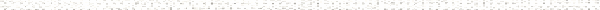An Engineering Statics Outline

 a. IntroductionObjective: State Newton's Laws and apply them to the study of staticsObjective: tate the importance of drawing a correct Free Body Diagram b. DesignObjective: State the three steps of the design process: Conceptual Design, Design Development, and Final DesignObjective: Explain the importance of proper statics analysis to the Development phase c. Problem SolvingObjective: Illustrate the problem solving process and adopt this process for all problem solutionObjective: State the six steps of the problem solving process.Objective: Explain the real-world importance of documenting the solution (i.e.,"showing your work") d. UnitsObjective: Use unit conversion to manipulate the units provided in a problem statement in order to get the desired output units.Objective: State the base units and abbreviations used in the US and S.I. systems.Objective: State the S.I. prefixes for positive and negative powers of ten up to 10^3.Objective: Recognize and name the letters of the Greek alphabet. e. Forces in StaticsObjective: Identify ten kinds of forces (five pairs)Objective: State the importance of correctly identifying loads on a structure. f. Mass vs. ForceObjective: State the units of mass and force in the US and S.I. systemsObjective: Define the unit kips.Objective: Calculate the force of gravity on an object of a given mass.Objective: Explain the difference between force and mass g. Algebra ReviewObjective: Solve one equation with one unknownObjective: Solve two equations with two unknownsObjective: Use substitution to solve algebraic equationsObjective: Use elimination to solve algebraic equations h. Trigonometry ReviewObjective: Use the definitions of sin, cos, and tan to find the sides and angles of right trianglesObjective: Use the law of sines, law of cosines, and law of tangents to solve polygonsObjective: Relate the Pythagorean Theorem to the Law of Cosines i. Geometry ReviewObjective: Use the principles of parallel lines to find equal, supplementary, and complementary angles.Objective: Given two adjacent interior angles on a triangle, find the exterior angle of the opposite point.Objective: Describe the result when a perpendicular is drawn from the hypotenuse to the opposite vertex.Objective: Explain what is meant by "similar triangles"Objective: Find the measure and sum of the interior and exterior angles on an n-sided polygon.Objective: Find the properties of an arc segment given the radius and included angle j. Significant DigitsObjective: State the rules of significant digits.Objective: Given a calculation involving numbers, correctly determine the number significant digits in the result.Objective: Discriminate between measurements and exact numbers.Objective: Discriminate between the rules for significant digits when adding & subtracting, and those when multiplying and dividing.Objective: Discriminate between accuracy and precision